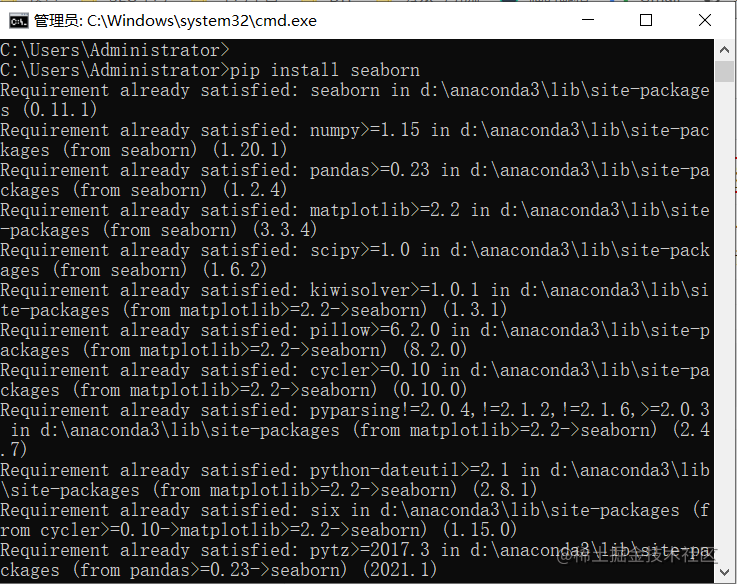﻿ Python 数据可视化之Seaborn详解_python_脚本之家
python# Python 数据可视化之Seaborn详解

## 安装

pip install seabornSeaborn 建立在 Matplotlib 之上，因此它也可以与 Matplotlib 一起使用。一起使用 Matplotlib 和 Seaborn 是一个非常简单的过程。我们只需要像之前一样调用 Seaborn Plotting 函数，然后就可以使用 Matplotlib 的自定义函数了。

```# 导包
import seaborn as sns
import matplotlib.pyplot as plt
import pandas as pd

# 读取数据库
# 画线图
sns.lineplot(x="sex", y="total_bill", data=data)
# 使用 Matplotlib 设置标题
plt.title('Title using Matplotlib Function')
plt.show()
```## 散点图

```# 导包
import seaborn as sns
import matplotlib.pyplot as plt
import pandas as pd
# 读取数据库
sns.scatterplot(x='day', y='tip', data=data,)
plt.show()
``````# 导包
import seaborn as sns
import matplotlib.pyplot as plt
import pandas as pd
# 读取数据库
sns.scatterplot(x='day', y='tip', data=data,
hue='sex')
plt.show()
```## 线图

Seaborn 中的 Line Plot 使用 `lineplot()` 方法绘制。 在这种情况下，我们也可以只传递 data 参数。

```# 导包
import seaborn as sns
import matplotlib.pyplot as plt
import pandas as pd

# 读取数据库
sns.lineplot(x='day', y='tip', data=data)
plt.show()
``````# 导包
import seaborn as sns
import matplotlib.pyplot as plt
import pandas as pd

# 读取数据库
# 仅使用数据属性
sns.lineplot(data=data.drop(['total_bill'], axis=1))
plt.show()
```## 条形图

Seaborn 中的条形图可以使用`barplot()`方法.

```# 导包
import seaborn as sns
import matplotlib.pyplot as plt
import pandas as pd

# 读取数据库
sns.barplot(x='day',y='tip', data=data,
hue='sex')
plt.show()
```## 直方图

Seaborn 中的直方图可以使用`histplot() `函数绘制。

```# 导包
import seaborn as sns
import matplotlib.pyplot as plt
import pandas as pd

# 读取数据库
sns.histplot(x='total_bill', data=data, kde=True, hue='sex')
plt.show()
```